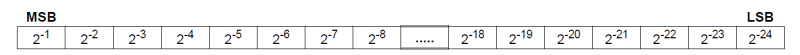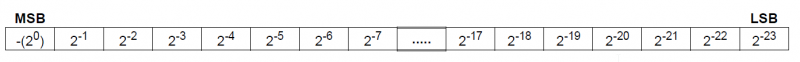# Optimizing odd data format conversion

I am reading SPI data from an IC that is represented in a fairly unique way. The register contains 24 bits of unsigned data in the range of 0 <= value <= 1. It is calculated using the following format:I’ve written the following function to take the returned data (which I first convert from a string of values to a 24 bit integer) and convert it into a usable squirrel float. I’ve gotten it tightened up to run in around 1.5ms (it was over 4.5ms before I tried to optimize it!) but I’d like for it to go even faster - any Electric Imp/Squirrel Guru’s have any recommendations?

`
function constrained24BitToFloat(regVal){
local retVal = 0.0;
local powIndex = -1;
local clock = hardware.micros.bindenv(hardware)
local pow = math.pow.bindenv(math)
local start = clock()

``````    for(local i=23; i >= 0; i--){
retVal += ((regVal >> i) & 0x01)*pow(2, powIndex--)
}

local end = clock()
server.log("Conversion time = " + (end-start))
return retVal
``````

}

server.log(constrained24BitToFloat(0x03A748)) //should print 0.0142713 in ~1.536ms

`

Fantastic - that runs in 0.012ms! I knew someone smart on the forums could help come up with something much more elegant.

If anyone is interested into why this works, it normalizes the integer (which is automatically converted to a float in the division) to the range 0-1. In other words 16777216 is the largest positive 32-bit float.

On the same device, some registers are stored as two’s complement in the range of -1 <= value <= 1. Using @DrJack’s recommendation, I’ve already got it down to running in 0.017ms, but I am now just curious if there is a better way to do things.`function constrained24BitTwosComplementToFloat(regVal){
local sign = 2;

``````local clock = hardware.micros.bindenv(hardware)
local start = clock()

if (regVal & 0x800000){
regVal = ((~regVal & 0xFFFFFF) + 1) //two's complement value
sign = -2;
}
regVal/16777216.0*sign
local end = clock()
server.log("Conversion time = " + (end-start))

return  regVal/16777216.0*sign
``````

}

server.log(constrained24BitTwosComplementToFloat(0x03A748)) //should return 0.0285425 in .017ms`

Any ideas?

EDIT: I pasted the wrong format - it’s been fixed.

1. Floating point math is hard (i.e. 16777216.0*sign)
2. In line if statements are ever so slightly more efficient than block statements
3. Variables are for chumps

I was able to get it down to .015 / 0.19 (2’s comp) from 0.017 / 0.22 (2’s comp):

`function constrained24BitTwosComplementToFloat(regVal){
local clock = hardware.micros.bindenv(hardware)
local start = clock();

``````// return statements commented out for timing
if (regVal & 0x800000) /* return */ ((~regVal & 0xFFFFFF) + 1)/-8388608.0;
else /* return */ regVal/8388608.0;

local end = clock();
server.log("Conversion time = " + (end-start));
``````

}

server.log(constrained24BitTwosComplementToFloat(0x03A748)) //should return 0.0285425 in ~.015 +/- 0.001ms
server.log(constrained24BitTwosComplementToFloat(0x83A748)) //should return -0.971457 in ~.019ms +/- 0.001ms`

The actual function (with timing code removed looks like this):

`function constrained24BitTwosComplementToFloat(regVal){
if (regVal & 0x800000) return ((~regVal & 0xFFFFFF) + 1)/-8388608.0;
else return regVal/8388608.0;
}

Side note: if you’re only using this one or two places, and timing is critical, I would hard code it. If you put the timing around the function, instead of inside of it, it takes orders of magnitude longer…

More neat things:

An average of 100,000 calculations results in:

Positive: 14.8271 ms
2’s Comp: 18.6957 ms

If we switch it to a ternary operator instead of an if statement (i.e. (regVal & 0x800000) ? ((~regVal & 0xFFFFFF) + 1)/-8388608.0 : regVal/8388608.0;), we get:

Positive: 14.5571 ms
2’s Comp: 18.7241 ms

Ever so slightly more efficient…

Thanks for all of the input - it’s always nice to learn something new (and see how much simpler my approach can be made…)!

Now that I see how compact the function can be made I’ll definitely consider hard coding it (I was already thinking that already to reduce the function overhead like you mentioned - I just wanted a digestible code snippet for the forums).

It is curious to me that the ternary operator is faster for positive numbers but slower for 2’s Comp. Assuming a uniform average of positive and negative values its faster overall but any idea why the speed up and slow down?

I’m also curious as to why in line if statements are ever so slightly more efficient than block statements - if there is only one instruction inside of an in line if, shouldn’t the byte code compiler optimize the instructions and remove the slightly slower block?

P.S. if there was an asynchronous version of the SPI peripheral where I could call these optimized data format conversions in sudo real time that would really make my day (and enable some really fast, gapless waveform captures using the Cirrus Logic CS5484)We’re talking about microsecond differences in an interpreted language. Those numbers will be slightly different every time you run it.

As for inline vs block statements - I’m not 100% sure why that happens (a question for @peter probably)… it’s just something that @aron noticed while writing very tight loops.

Squirrel integers are inherently two’s-complement; if you promote your sign bit into the integer’s sign bit, you don’t need the “if”:
`return (regVal<<8)/2147483648.0; // works for positive or negative`
And in fact, floating-point multiplication is sometimes fractionally faster than division:
`return (regVal<<8)*4.656612873077393e-10`
where that float constant equals 1.0/2147483648.0.

More elaborate schemes involving constructing the IEEE754 float representation as an integer and using casti2f(), probably cost more in Squirrel than they save in maths.

Peter

if there is only one instruction inside of an in line if, shouldn’t the byte code compiler optimize the instructions and remove the slightly slower block?

The current Squirrel compiler is fairly simple, which makes it fast. For our purposes that’s not always the trade-off we’d like – our users often want more performance and/or better code-density and don’t much care how long the compilation takes. Making the Squirrel compiler a bit smarter is an active research project here at Electric Imp…

Peter